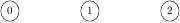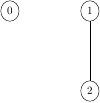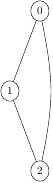Queries for Graphs: search statistic / browse statistics / browse maps from / browse maps to

# Definition & Example

• A (finite, undirected, simple) graph $G = (V,E)$ consists of a finite set $V$ of vertices and a set $E \subseteq \binom{V}{2}$ of edges.

• Two graphs $G = (V,E)$ and $G = (V',E')$ are isomorphic if there is a bijection $\psi : V \rightarrow V'$ such that

$$\{u,v\} \in E \Leftrightarrow \{\psi(u),\psi(v)\} \in E'.$$

• Unlabelled graphs on $n$ vertices are graphs up to graph-isomorphism.

• A canonical form of an unlabelled graph is a relabelling of the vertices (an isomorphic graph) such that any two graphs are isomorphic if and only if they have the same canonical form.

 the 4 Graphs of size 3([],3) ([(1,2)],3) ([(0,2),(1,2)],3) ([(0,1),(0,2),(1,2)],3)

• Unlabelled graphs are graphically represented by their unlabelled vertices with edges connecting adjacent vertices.

• For the number of unlabelled graphs see OEIS:A000088.

# Further definitions

• If $\{u,v\}$ is an edge in a graph $G$, then $u$ and $v$ are adjacent vertices. $u$ and $v$ are also known as neighbors. The set of neighbors of $v$, denoted $N(v)$, is called the neighborhood of $v$. The closed neighborhood of $v$ is $N[v]=N(v)\cup {v}$.

• If two edges share a vertex in common (e.g. $\{u,v\}$ and $\{v,w\})$, then they are adjacent edges.

• The degree of a vertex $v$, denoted deg($v$), is the number of vertices adjacent to $v$.

• We call $|V(G)|$, the cardinality of the vertices of a graph $G$, the order of the graph. We also say $|E(G)|$, the cardinality of the edges of a graph $G$, is the size of the graph.

• A graph of size 0 is called an empty graph. Any graph with at least one edge is called nonempty.

• A graph is complete when any two distinct vertices are adjacent. The complete graph of $n$ vertices is notated $K_{n}$

• A planar graph is a graph that can be embedded in the plane. I.e. it can be drawn on the plane such a way that its edges intersect only at their endpoints.

• A walk $W$ in a graph $G$ is a sequence of vertices in $G$, beginning at a vertex $u$ and ending at a vertex $v$ such that the consecutive vertices in $W$ are adjacent in $G$.

• A walk whose initial and terminal vertices are distinct is called an open walk, otherwise it is a closed walk.

• A walk in which no edge repeats is called a trail.

• A path $P$ in a graph $G$ is a sequence of edges which connect a sequence of vertices which, are all distinct from one another. A path can also be thought of as a walk with no repeated vertex.

• A simple path is one which contains no repeated vertices (in other words, it does not cross over itself).

• If there is a path from a vertex $u$ to a vertex $v$ then these two vertices are said to be connected. If every two vertices in a graph $G$ are connected, then $G$ is itself a connected graph.

• A nontrivial closed walk in a graph $G$ in which no edge is repeated is a circuit in $G$.

• A circuit with vertices $v_1, v_2, ..., v_k, v_1$ where $v_2, ..., v_k$ are all distinct is called a cycle.

• Let $G$ be a nontrivial connected graph. A circuit $C$ of $G$ that contains every edge of $G$ (necessarily exactly once) is called an Eulerian Circuit. Any graph which contains an Eularian Circuit is called Eularian. A graph is Eulerian if and only if all its vertices have even degrees.

A nontrivial connected graph $G$ is Eularian if and only if every vertex of G has even degree.

• Every planar graph is four-colorable. That is, the chromatic number of a planar graph is at most four.

• wikipedia

• G. Chartrand, L. Lesniak, and P. Zhang. Graphs and Digraphs. CRC Press, Oct. 2010.

# Technical information for database usage

• A graph is uniquely represented as a tuple (E,n) where E is the sorted list of edges in the canonical labelling and n is the number of vertices.
• Graphs are graded by the number of vertices.
• The database contains all graphs of size at most 7.

If you want to edit this wiki page, you can download the raw markdown and send your new version to info@findstat.org